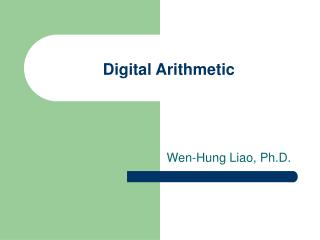Download PresentationDigital Arithmetic

Loading in 2 Seconds...

# Digital Arithmetic - PowerPoint PPT Presentation

Digital Arithmetic. Wen-Hung Liao, Ph.D. Objectives. Perform binary addition, subtraction, multiplication, and division on two binary numbers. Add and subtract hexadecimal numbers. Know the difference between binary addition and OR addition.I am the owner, or an agent authorized to act on behalf of the owner, of the copyrighted work described.
Download Presentation## Digital Arithmetic

An Image/Link below is provided (as is) to download presentation

Download Policy: Content on the Website is provided to you AS IS for your information and personal use and may not be sold / licensed / shared on other websites without getting consent from its author.While downloading, if for some reason you are not able to download a presentation, the publisher may have deleted the file from their server.

- - - - - - - - - - - - - - - - - - - - - - - - - - E N D - - - - - - - - - - - - - - - - - - - - - - - - - -
Presentation Transcript
1. Digital Arithmetic Wen-Hung Liao, Ph.D.

2. Objectives • Perform binary addition, subtraction, multiplication, and division on two binary numbers. • Add and subtract hexadecimal numbers. • Know the difference between binary addition and OR addition. • Compare the advantages and disadvantages among three different systems of representing signed binary numbers. • Manipulate signed binary numbers using the 2's complement system. • Understand the BCD adder circuit and the BCD addition process. • Describe the basic operation of an arithmetic/logic unit.

3. Objectives (cont’d) • Employ full adders in the design of parallel binary adders. • Cite the advantages of parallel adders with the look-ahead carry feature. • Explain the operation of a parallel adder/subtractor circuit. • Use an ALU integrated circuit to perform various logic and arithmetic operations on input data. • Read and understand the IEEE/ANSI symbol for a parallel adder. • Analyze troubleshooting case studies of adder/subtractor circuits. • Program a PLD to operate as a 4-bit full adder.

4. Binary Addition • Performed in the same manner as the addition of decimal numbers. • Most important arithmetic operation in digital systems, since subtraction, multiplication and division are all based on addition.

5. Representing Signed Numbers • Sign-magnitude system: left most bit as sign bit (0 for +, 1 for -), remaining bits as the magnitude. • Problems: • How to perform addition? • Two zeros: 1 0000 and 0 0000

6. 1’s and 2’s-Complement Form • 1‘s complement: change 0 to 1 and 1 to 0. • 2’s complement: take 1’s complement and add 1 to the LSB. • Examples: +13, -9,+3,-2,-8 • Negation vs. complement

7. 2’s Complement • Range of values can be represented using 1 sign bit and N magnitude bits:-2^N to 2^N-1 • 1000 = -2^3 =-8 • 10000 = -2^4 = -16…

8. Addition in 2’s Complement Form • Case I: Two positive numbers • Case II: Positive number and smaller negative number • Case III: Positive number and larger negative number • Case IV: Two negative numbers • Case V: Equal and opposite numbers

9. Subtraction in 2’s Complement • A – B = A + (-B) • Arithmetic overflow: results of addition or subtraction fall outside the range of values that can be represented.

10. Binary Multiplication • Similar to multiplication of decimal numbers • 1001 x 1011 • What about the sign? • Overflow?

11. Binary Division • 1001 divided by 11

12. BCD Addition • Sum equals 9 or less: digit-by-digit addition • Sum greater than 9: • Example: 6 + 7 • Add 6 (0110) to correct the result (will produce a carry)

13. Hexadecimal Arithmetic • Hex addition • Hex subtraction • Convert to binary,take 2’s complement, convert back to Hex • Subtract each hex digit from F, then add 1 • Hex representation of signed numbers: • 3A  +58 • E5  -29 • When MSD >=8, negative

14. Arithmetic Circuits • Parallel Binary Adder (Figure 6-5*): sum and carry bit.

15. Design of a Full Adder • Figure 6-6 (Truth Table) • Figure 6-7* • Half adder: take 2 inputs and generate sum and carry bits.

16. Four-Bit Parallel Adder • Complete parallel adder with registers (Figure 6-9):

17. Register Notation • Register notation: [A]: the content of register A • Example: [A]=1011 means that A3=1, A2=0, A1=1, A0=1.

18. Carry Propagation • For parallel adders, sum bit generated in the last position (MSB) depended on the carry that was generated by the addition in the first position (LSB). • More delay for addition of 32 or 64 bit numbers. • Use look-ahead carry to reduce propagation delay.

19. Integrated-Circuit Parallel Adder • 4-bit parallel adder: 74HC283 • Cascading parallel adders

20. 2’s Complement System • Figure 6.11: addition (C0=0) • Figure 6.12: subtraction (C0=1)

21. Combined Addition and Subtraction • Figure 6-13

22. BCD Adder • How to detect when sum > 9? • X=S4+S3(S2+S1) • Figure 6-14

23. Cascading BCD Adders

24. ALU Integrated Circuits

25. ALU ICs • 74LS382/74HC382 • CLEAR, B minus A, A minus B, A plus B, A XOR B, A+B, AB, preset • Expanding the ALU: combining 2 4-bit ALUs. • IEEE symbols